字节跳动（今日头条）推荐算法实习生面试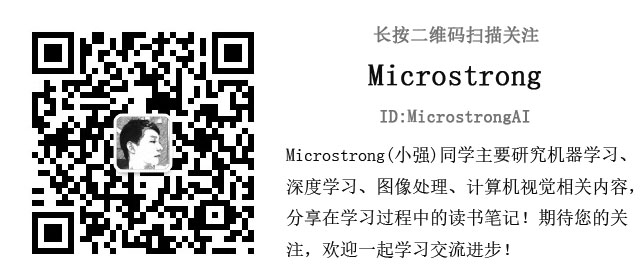2018-05-16 17:00 一面：

（1）自我介绍。

（2）介绍自己是如何去除水印和增加水印安全性的工作，对于自己做过的项目问的很具体。

（3）让详细介绍一下逻辑回归，包括逻辑回归的分类公式，损失函数、逻辑回归分类的过程。

（4）问了一下逻辑回归中损失函数的作用？

（5）逻辑回归中除了损失函数能衡量模型的好坏，还有没有其他的方法？

（6）接下来，重点来了！字节跳动中传说的手撕代码来了！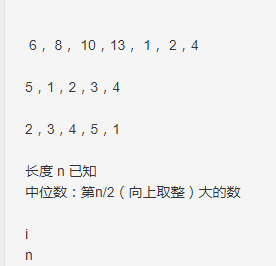原数组：
6,8,10,13,1,2,4

1，2,4,6,8,10
#############################

5,1,2,3,4,

1,2,3,4,5
##############################

2,3,4,5,1

1,2,3,4,5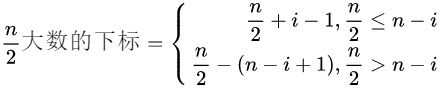1.如果你的第n/2大的数在断点后边，那么可以直接通过下标计算得出第n/2大的数的下标。下标计算公式如下：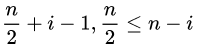2.如果你的第n/2大的数在断点的前面，那么你可以把断点后边小于第n/2大的数给算上，然后在往前面查找到第n/2大的数。下标计算公式如下：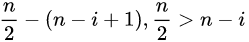public class Main {

public static void main(String[] args){
//	    int[] array = {6, 8, 10, 13 , 1, 2, 4};
//		int[] array = {5, 1, 2, 3, 4};
int[] array = {2, 3, 4, 5, 1};
int n = array.length;
int location = (int) Math.ceil(n/2.0);
int index = searchIndex(array);
int temp = 0;
if(location <= n - index){
temp = array[index+location - 1];
}else{
temp = array[location-(n-index+1)];
}
System.out.print(temp);
}

public static int searchIndex(int[] array){
for(int i=0; i<array.length; i++){
if(array[i] > array[i+1]){
return i + 1;
}
}
return 0;
}

}	

（1）我们要找数组中最小数的下标，那么我们前提是不知道最小数是多少，如何进行比较查找最小数。

（2）在折半查找的过程中，如何确定最小数是在左半边还是在右半边，换句话说，你是搜索左边的数组还是搜索右边的数组呢！

public class Main {

public static void main(String[] args){
int[] array = {6, 8, 10, 13 , 1, 2, 4};
//		int[] array = {5, 1, 2, 3, 4};
//		int[] array = {2, 3, 4, 5, 1};
int n = array.length;
int location = (int) Math.ceil(n/2.0);
int index = Select_k(array, 0, n-1, location);
System.out.print(index);
}

public static int quickSortOneTime(int[] array, int low, int high){ //一趟快速排序
int  key = array[low];
while(low < high){
while(key < array[high] && low < high)  high--;
array[low] = array[high];
while(key > array[low] && low < high)   low++;
array[high] = array[low];
}
array[high] = key;
return high;
}

public static int Select_k(int[] array, int low, int high, int k) {
int index;
if(low == high) return array[low];
int partition = quickSortOneTime(array, low, high);
index = high - partition + 1;  //找到的是第几个大值
if(index == k) {
return array[partition];
}else if(index < k) {//此时向左查找
return Select_k(array, low, partition-1, k-index);  //查找的是相对位置的值，k在左段中的下标为k-index
}else {
return Select_k(array, partition+1, high, k);
}
}

}